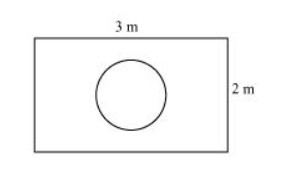# Suppose you drop a die at random on the rectangular region shown in the given figure.

Question:

Suppose you drop a die at random on the rectangular region shown in the given figure. What is the probability that it will land inside the circle with diameter 1 m?Solution:

Area of rectangle $=1 \times b=3 \times 2=6 \mathrm{~m}^{2}$

Area of circle (of diameter $1 \mathrm{~m})=\pi r^{2}=\pi\left(\frac{1}{2}\right)^{2}=\frac{\pi}{4} \mathrm{~m}^{2}$

$P($ die will land inside the circle $)=\frac{\frac{\pi}{4}}{6}=\frac{\pi}{24}$